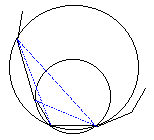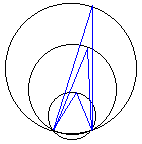# Separating point sets with a circle

### Problem

Let there be given 2n + 3 points (n > 0) such that no 4 lie on the same circle. Prove that it's possible to select 3 points so that the circle passing through these points contains in its interior exactly n points from the set whereas the remaining n points lie in its exterior.

### Solution

Let's use the notion of convex hull that served us so well so many times before. Thus, let's enclose our set into its convex hull. We should start somewhere looking for our three points. Choose any side of the convex hull. This gives two points. Fix these two. Selecting every time a new point in addition to these two, we can draw (2n+1) circles because no 4 points belong to the same circle. These circles are naturally ordered. Can't we use this order?But what is it, to start with? What can we say about it? Do all circles have different radii? No, it must not necessarily be true. (To see this, start with two intersecting circles of equal radius. Besides the two points of intersection, we may choose three additional points: one on each of the circles and one elsewhere. This a counterexample.) Recollect now that, in addition to the two fixed points, every circle contains exactly one point from the given set. Connecting this point with the fixed two determines an angle. The angle under which the fixed segment is seen from the selected point. For a fixed circle, this angle does not depend on the exact location of the point on the circle. Therefore, it's rather a circle related than a point related. Might it be a candidate to determine the order of the circles?Yes, indeed. As the angles become smaller so the circles (or rather their part above the two fixed points) expand. The circle with the largest angle contains no points inside it. The next one contains the point that defined the previous circle. The next one contains this point too but, in addition, it also contains the point that defines the second circle, and so on. The circle number (n+1) contains exactly n points inside and n points outside itself.

Note that the solution may not be unique because it depends on the original selection of two fixed points.

Richard Beigel sent me the following simple proof:

Let there be a set S of N points. For each pair of points in S, draw its perpendicular bisector. Let p be any point in the plane that is not on any of those perpendicular bisectors. Then no two points in S are equidistant from p. Let the distances from p to each point in S be d1, d1, ..., dN. Relabel the points so that 0 = d1 < d2 < ... < dN. Then, for any k > 0, the circle with center p and radius (dk + dk+1)/2 separates some k from the remaining N - k points.

Richard's result allows 4 or more points to lie on the same circle. Returning to the original problem, N = 2n + 3, we first find a circle that separates n points from the remaining n + 3. Then we start expanding it until it first touches at least one of the n + 3 points. If 3 points lie on the circle, t's all finished. If only 1 or 2 points lie on the circle we continue extending it keeping the "acquired" points on the circle until there are exactly three points there (it can't be more by the conditions of the problem.)

Richard also sent another proof that made use of less elementary mathematics and the following variation on the first proof.

Consider pairwise distances of the given 2n + 3 points and select two points A and B such that dist(A,B) is the shortest. Consider a pencil of circles (i.e., all circles) through these two points. Start with a circle whose diameter is AB. Move the center along the perpendicular bisector of AB, starting from the midpoint, out to infinity in one direction, and back in from infinity in the other direction. As the circle crosses points in S, the number of points inside/outside the circle progresses as follows.

 inside 0 1 2 ... k ... (2n + 1)-(k + 1) (2n + 1) - (k + 2) ... 0 outside 2n+1 2n 2n - 1 ... (2n + 1) - k ... k + 1 k + 2 ... 2n + 1

Assume there are k+1 points on one side of the line AB, (2n + 1)-k on the other. The three stars indicate marks where the center goes to infinity and back around the other side. Interchanging inside/outside after infinity is reached, you see the progression (0, 2n + 1), ..., (2n + 1, 0) so at some point there were n points on each side of the circle with one addtional point on the circle.Copyright © 1996-2018 Alexander Bogomolny

 64856139